/

### The generalised gamma distribution

The generalised gamma (or generalized gamma) distribution is a generalisation of the gamma distribution that includes several of the parametric distributions typically used in survival analysis.

 Distribution name Generalised gamma distribution Common notation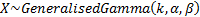Parameters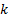= shape parameter (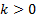)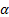= shape parameter (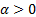)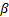= scale parameter (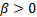) Domain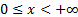Probability density function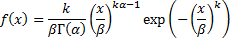Cumulative distribution function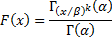Mean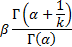Variance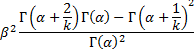Skewness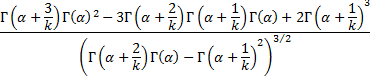(Excess) kurtosis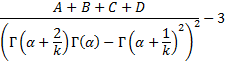where: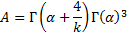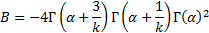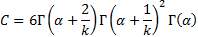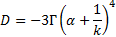Other comments Its non-central moments are: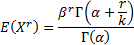The Weibull distribution is a special case with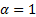. The gamma distribution is a special case with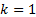.

Nematrian web functions

Functions relating to the above distribution may be accessed via the Nematrian web function library by using a DistributionName of “generalised gamma”. Functions relating to a generalised version of this distribution including an additional location (i.e. shift) parameter may be accessed by using a DistributionName of “generalised gamma4”, see also including additional shift and scale parameters. For details of other supported probability distributions see here.